# Annulus Problems

Annulus is a ring-shaped object, a region bounded by two concentric circles.

#### Number of problems found: 29

• The collar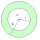The collar on the dress has the shape of an annulus 6 cm wide. The circumference of the inner circle is 31.4 cm. How much cm2 of fabric is needed to make one collar?
• Round skirtThe cut on the round skirt has the shape of an annulus. Determine how much m² of fabric will be consumed on an 80 cm long skirt. The circumference of the waist is a circle with a smaller radius and is 69 cm.
• Brass sphereFind the weight of a brass ball with an outer radius of 12 cm, a wall thickness of 20 mm if the brass's density is 8.5 g/cm3.
• The hollow cylinderThe hollow cylinder has a height of 70 cm, an outer diameter of 180 cm, and an inner diameter of 120 cm. What is the surface of the body, including the area inside the cavity?
• Annulus from triangleCalculate the content of the area bounded by a circle circumscribed and a circle inscribed by a triangle with sides a = 25mm, b = 29mm, c = 36mm
• Round flowerbed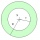Around a round flowerbed with a diameter of 6 meters and I will make a sidewalk up to 0.5 meters wide. How much gravel is needed if the layer is to be 5 cm high?
• AnnulusTwo concentric circles with radii 1 and 9 surround the annular circle. This ring is inscribed with n circles that do not overlap. Determine the highest possible value of n.
• Steel tubeThe steel tube has an inner diameter of 4 cm and an outer diameter of 4.8 cm. The density of the steel is 7800 kg/m3. Calculate its length if it weighs 15 kg.
• Annular areaThe square with side a = 1 is inscribed and circumscribed by circles. Find the annular area.
• Mice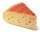Mice consumed a circular hole in a slice of cheese. The cheese has the shape of a circular cut with a radius of 20 cm and an angle of 90 degrees. What percentage of the cheese ate mice if they made 20 holes with a diameter of 2 cm?
• Metal tubeCalculate the metal tube mass 8dm long with the outer radius 5cm and the inner radius 4.5cm and 1cm3 of this metal is 9.5g.
• Concentric circlesThere is given a Circle K with a radius r = 8 cm. How large must a radius have a smaller concentric circle that divides the circle K into two parts with the same area?Marie wants to make a cone-shaped witch's hat for a masquerade ball. How much material will it need if it counts on an annular rim with diameters of 28cm and 44cm? Hat side length is 30cm. Add 5% of the material to the bust. Round to cm2.
• Circle annulusThere are 2 concentric circles in the figure. Chord of larger circle 10 cm long is tangent to the smaller circle. What are does annulus have?
• Around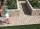Around the circular flowerbed with a radius of 2 m is a sidewalk 80 cm wide. How many square meters does the sidewalk have?
• The coilHow many ropes (the diameter 8 mm) fit on the coil (threads are wrapped close together) The coil has dimension: the inner diameter 400mm, the outside diameter 800mm and the length of the coil is 470mm
• Two annulusesThe area of the annular circle formed by two circles with a common center is 100 cm2. The radius of the outer circle is equal to twice the radius of the inner circle. Determine the outside circle radius in centimeters.
• Pipe cross sectionThe pipe has an outside diameter 1100 mm and the pipe wall is 100 mm thick. Calculate the cross section of this pipe.
• AnnulusThe radius of the larger circle is 8cm, the radius of smaller is 5cm. Calculate the contents of the annulus.
• The pipe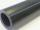The pipe is 1.5 m long. Its outer diameter is 60 cm, inner diameter is 52 cm. Calculate the pipe's weight if the material's density from which it is made is 2 g/cm3. Round the results to whole kilograms.

Do you have an exciting math question or word problem that you can't solve? Ask a question or post a math problem, and we can try to solve it.

We will send a solution to your e-mail address. Solved examples are also published here. Please enter the e-mail correctly and check whether you don't have a full mailbox.

Please do not submit problems from current active competitions such as Mathematical Olympiad, correspondence seminars etc...